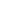Original research

# Analysis and Comparison for Prediction of Diabetic among Pregnant Women using Innovative K-Nearest Neighbor Algorithm over Logistic Regression with Improved Accuracy

## Abstract

Aim: In comparison to the K-Nearest Neighbor(KNN)algorithm, Logistic Regression(LR) was used in machine learning algorithms for the prediction of diabetes among pregnant women to get better accuracy, PEARCEsensitivity, and precision. Materials and methods: To verify the usefulness of the technique, researchers collected data sets from free available data sets such as the Pima Indian dataset from the UCI website to examine diabetes among pregnant women. There are two groups in this study: K-Nearest Neighbor (N=20) and Logistic Regression (N=20), each having a sample size of 40. A pre-test power of 80%, a threshold of 0.05, and a confidence interval of 95% are used in the sample size calculation. Results: The accuracy, sensitivity, and precision of algorithms are used to evaluate their performance. K-Nearest Neighbor (KNN) accuracy rate is 72.44 percent, but Linear Regression (LR) accuracy is 76.67%. The sensitivity rate for K-Nearest Neighbor is 74.42 percent, while the sensitivity rate for Linear Regression (LR) is 76.16 percent. The precision rate for K-Nearest Neighbor (KNN) is 73.75percent, whereas the precision rate for Linear Regression(LR) is 81.87 percent. The accuracy rate is significantly different P=0.366(P>0.05). Conclusion: When compared to the Innovative K-Nearest Neighbor algorithm, the Logistic Regression algorithm predicts better classification in discovering the accuracy, sensitivity, and precision for accessing the rate for prediction of diabetes among pregnant women.

## Imprint

Venkata Sai Kumar Pokala, Neelam Sanjeev Kumar. Analysis and Comparison for Prediction of Diabetic among Pregnant Women using Innovative K-Nearest Neighbor Algorithm over Logistic Regression with Improved Accuracy. Cardiometry; Issue 25; December 2022; p.949-955; DOI: 10.18137/cardiometry.2022.25.949955; Available from: https://www.cardiometry.net/issues/no25-december-2022/k-nearest-neighbor-algorithm

## Keywords

Diabetes prediction,   Innovative K-Nearest Neighbor algorithm,  Logistic Regression algorithm,  Artificial Intelligent,  AccuracyCurrent issueCardiometry's libraryFounders of Cardiometry